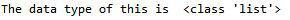# Sample of a Python User Defined Function

In the following sample, we are going to talk about Python user defined function. A function in Python is an object that takes arguments and returns a value. A function is a block of organized, reusable code that is used to perform a specific task. Functions provide better modularity of the application and significantly increase the level of code reuse. There are some rules for creating functions in Python. You will learn about these rules if you read through the following sample.

We advise all students who are starting to study programming to read it through. Also, you can find examples of completed assignments in other disciplines. After you read our sample, you will be able to work with user defined functions in Python. You will see that such functions can be easily created by you. So, don’t wait anymore and read the following example – it will surely be helpful for you.

Python Functions

In this particular paper we are going to discuss general structure of user-defined functions in Python, along with the overview of the most interesting built-in ones.

User-defined function

In Python, in order to define some function, you just need to write ​def ​and the name of the function. It is very simple. The only thing that should be followed strictly is indentation, because in Python there are no braces that specify the beginning and ending of the method. You just need to keep your code in the appropriate place to avoid errors.

```def simpleOne(val):
for i in range(val):
print("It's a new day")

simpleOne(​4​)
```

Output:

```"It's a new day"
"It's a new day"
"It's a new day"
"It's a new day"
```

This program just outputs string for as many times as you specify in its parameter.

As you see, the function is called by simply writing its name with the parameter if there is one.

Built-in functions

The first built-in function that appears when learning Python is ​print(​). ​It simply outputs the contents of what is inside this method. Even the example above uses it.

In the next example, two functions are used: ​list()​​ and ​zip()​. ​The first returns the list of separate items depending on what is in the arguments list in the parentheses. Zip() method combines items from several tuples by their index.

```a = ['Sam', 'Dean']
b = [1992, 2013]
c = [1, 2, 3, 4, 5]
print(list(zip(a, b, c)))
```

Output:

```[('Sam', 1992, 1), ('Dean', 2013, 2)]
```

In this example we are integrating all the existing tuples, and since there can only be two mutual pairs, you can observe them in output.

```a = ['Sam', 'Dean']
b = [1992, 2013]
c = [1, 2, 3, 4, 5]
for l,m in zip(a, b):
d[l]=m
form = l + ":{ " + l + "}"
print(form.format(**d))
```

Output:

```"Sam: 1992"
"Dean: 2013"
```

In the next example several functions are used at the same time: ​input()​, ​split()​,​ range()​, len()​.

Input method is for accepting user types in console. Split function is for separating some string with the specified in the arguments symbol or character, thus creating more independent units. Range(), in this example, is used for setting the upper limit of loops. Len() returns the size of some variable that is in the parentheses.

All in all, this small program receives input from the user, separates it by blank space, and then outputs each individual value on the new line.

```print("Enter some words and numbers")
s = input()
some = s.split(" ")
for i in range((len(some))):
print(some[i])
```

You might wonder what is the data type of that variable ‘some’. You can guess or use the function type() to find out for sure. So, if we add one line of code to the above line, what we receive is:```print("The data type of this is ", type(some))